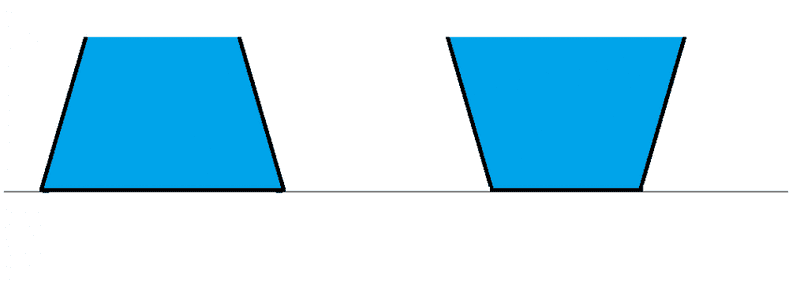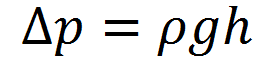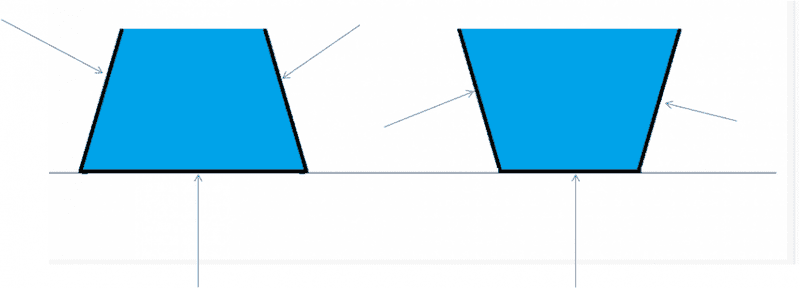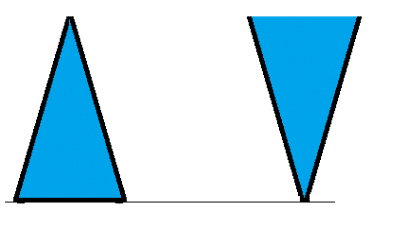# Pressure is equal or force is equal at the bottom?

• Ravi Singh choudhary
Hopefully, everyone agrees that the two systems weigh the same. However,...In summary, pressure is equal at the bottom of both containers, and the total upward force on the bottom in terms of pressure is the same.f

#### Ravi Singh choudharyProblem Statement:
Both containers are just equal in volume and having same height; you can assume one is upside down of another. What is pressure at the bottom of the containers. Let's say left side is container 1 and one on right side is 2.

Relevant equation:h is the depth of fluid from the top surface of fluid.

My attempt:
Thought 1
: Pressure will be equal at bottom for both the cases; and force to the bottom we can find with multiplying the respective bottom surface areas.

Thought 2: As volume and density of both the liquid is same; so the mass. When we draw free body diagram of the liquid, it appears the forces will be equal at bottom will be equal not the pressure.

#### Attachments

I like your second thought. What is the total upward force on the bottom in terms of the pressure?

I like your second thought. What is the total upward force on the bottom in terms of the pressure?

Let's try this; I am connecting piezometer to the both tank near bottom sideways. What will be the level of liquid in the piezometer; it is same or different.

Last edited:
For a fluid at or near equilibrium, pressure in any horizontal plane (actually, a surface of equal g) will be equal. What that will be depends on the weight (force). Consider a nail (spike) and a 20 kg weight. Which would you prefer: 1). The weight on top of the spike on top of your toe OR 2) the spike on top of the weight on top of your toe? Would you argue that it makes no difference to your toe?

View attachment 101427
My attempt:
Thought 1
: Pressure will be equal at bottom for both the cases; and force to the bottom we can find with multiplying the respective bottom surface areas.
Yes.

Thought 2: As volume and density of both the liquid is same; so the mass. When we draw free body diagram of the liquid, it appears the forces will be equal at bottom will be equal not the pressure.
When you draw the free body diagram of the fluid, don't forget the forces on the fluid exerted by the sloping sides.

•Vibhor, Chestermiller and CWatters
For a fluid at or near equilibrium, pressure in any horizontal plane (actually, a surface of equal g) will be equal. What that will be depends on the weight (force). Consider a nail (spike) and a 20 kg weight. Which would you prefer: 1). The weight on top of the spike on top of your toe OR 2) the spike on top of the weight on top of your toe? Would you argue that it makes no difference to your toe?
I am not getting what you are saying.

Yes.

When you draw the free body diagram of the fluid, don't forget the forces on the fluid exerted by the sloping sides.
Weight of bodies are equal, so the forces. How pressure can be equal.

Weight of bodies are equal, so the forces. How pressure can be equal.
Yes, the weight of the fluid is the same in each container. When you speak of force, what particular force are are you referring to?

Weight of bodies are equal, so the forces. How pressure can be equal.
See post #5.

For the right hand vessel the force acting on the base is NOT just the pressure multiplied by the area of the base. There is also pressure acting vertically on the sloping sides and these also press on the base.

•Ravi Singh choudhary
I agree with TSny. In your force analysis, you neglected to include the upward and downward forces exerted on the fluid by the sloping sides. Don't forget that pressure acts perpendicular to all surfaces.

I agree with TSny. In your force analysis, you neglected to include the upward and downward forces exerted on the fluid by the sloping sides. Don't forget that pressure acts perpendicular to all surfaces.

Right. But in the left-hand case, the force on the sides points up and out, but does NOT make the whole thing weigh less!

I think there is a difference between the case where the container has sides only (but no bottom) and the container with rigid sides and bottom. In the former case the force on the table under the liquid is just area times pressure; in the latter case, it is the weight of the water plus container.

Right. But in the left-hand case, the force on the sides points up and out, but does NOT make the whole thing weigh less!

I think there is a difference between the case where the container has sides only (but no bottom) and the container with rigid sides and bottom. In the former case the force on the table under the liquid is just area times pressure; in the latter case, it is the weight of the water plus container.
Hopefully, everyone agrees that the two systems weigh the same. However, I believe some of the confusion is coming from not specifying clearly the "force" that one is talking about. There are at least three distinct "forces at the bottom" :

(1) The force that the fluid exerts against the flat bottom of the container
(2) The force that the flat bottom of the container exerts on the fluid
(3) The force that the flat bottom of the container exerts on the table

In post #8, I was trying to get the OP to clarify his use of the word "force".

Right. But in the left-hand case, the force on the sides points up and out, but does NOT make the whole thing weigh less!
Hi Ray. I'm a little confused, but I think we are saying the same thing. In the left-hand figure, the sides are pushing down and in on the fluid, and this downward component adds to the weight of the fluid, so a larger area is required at the base to push up on the fluid. In the right-hand figure, the sides are pushing up and in on the fluid, so a smaller area is required at the base to support the weight of the fluid. So, in the left-hand figure, the upward pressure of the base produces a force which is greater than the weight of the fluid, and, in the right-hand figure, the upward pressure of the base produces a force which is less than the weight of the fluid. In both cases, the weight of the fluid is the same.

This is a really good problem. My 2 cents, pressure is isotropic (independent of direction) and both thought 1 and 2 of the OP are correct. Now, while pressure is isotropic the force on the walls of the container are along the normal to the surface. Draw a free body that includes these forces.

This is a really good problem. My 2 cents, pressure is isotropic (independent of direction) and both thought 1 and 2 of the OP are correct. Now, while pressure is isotropic the force on the walls of the container are along the normal to the surface. Draw a free body that includes these forces.
Thought 2 is not correct. Several responders have pointed out that thought 1 is correct. Both the force and the pressure exerted by the base on the fluid cannot be the same in the two figures. It is the pressure that is the same in the two figures, not the force.

A free body diagram is always correct provided all the forces are correctly represented and the body in question is known. There are forces applied to the fluid by the container. There are forces applied to the container. The force of the ground on the container must counter the weight. This does have to equal the net forces applied by the water to the inside of the container which includes the side walls.

A free body diagram is always correct provided all the forces are correctly represented and the body in question is known. There are forces applied to the fluid by the container. There are forces applied to the container. The force of the ground on the container must counter the weight. This does have to equal the net forces applied by the water to the inside of the container which includes the side walls.
You do realize that the OP's original question was not about the force that the ground exerts on the container, right?

"You do realize that the OP's original question was not about the force that the ground exerts on the container, right?"

yes

The integral of the force of the water on the inside of the container is equal to the downward weight. The pressure times the area is the normal force on each area element. The pressure is given by the equation the OP quoted which yields the same value in both cases.

The pressure on the outside of the container is the weight divided by the area.

Weight of bodies are equal, so the forces. How pressure can be equal.

An upward force is applied to the bottom of each container originating from the pressure of the fluid. The point of the problem is possibly that one should just apply the laws of fluids and not worry too much about the seeming paradox caused by the identical weights and differing areas. Now, having noticed that the pressure applied by the ground is in fact different you can resolve this by understanding how the slanted sidewalls effect each case. The original problem says "pressure at the bottom". This could be taken both ways as inside and out. These are not equal given a rigid container.

Here is the FBD of fluid only; neglect the black lines resembling the wallsI am very much satisfied with TSny; When I would put weighing machine below the container; I would have same weight for both the container.

My thought is; I would connect a piezometer to the both of the container, it would have same fluid column height that means same pressure; since childhood we are reading pressure is scalar and independent of shape of the container. Horizontal component of forces of due to side walls will be nullified in both the cases.

What is the difference between two condition is the component of force that is only acting to the flat bottom. The net force on bottom is same for both the cases.

Moral of the story: net force to the bottom and pressure at bottom will be equal, the difference is the normal force acting only to the flat bottom.

My thought is; I would connect a piezometer to the both of the container, it would have same fluid column height that means same pressure; since childhood we are reading pressure is scalar and independent of shape of the container. Horizontal component of forces of due to side walls will be nullified in both the cases.

What is the difference between two condition is the component of force that is only acting to the flat bottom. The net force on bottom is same for both the cases.
I want to make sure I follow what you are saying. So let's take a numerical example. See diagram attached below, which shows numerical values for the top and bottom areas of the container as well as the height and volume of the container.

Take the density of the fluid to be 1000 kg/m3 and g = 10 m/s2. Atmospheric pressure is just a distraction, so let’s set atmospheric pressure to zero (we can always add that back in later, if we want). Also, neglect the weight of the empty container. Assume the container sits at rest on a table.

For the orientation shown in figure (a), find

(i) fluid pressure at the bottom of the container

(ii) force that the fluid exerts on the flat bottom of the container

(iii) force that the flat bottom of the container exerts on the table.

Repeat for the orientation shown in figure (b).
.

#### Attachments

Last edited:
•Ravi Singh choudhary, conscience and Chestermiller
Here are my observations for this setup .

1) The liquid pressure at the bottom of the two containers will be same , but the force exerted by liquid on the flat bottom would be different . Higher in case a) as compared to b) . By Newton's III law the flat bottom would be exerting a higher force in the first case .

2) If the two containers were placed on weighing scales , then the reading would be same .

The interesting part (for me ) is that even though liquid is exerting higher force on the flat bottom in the former case , the table beneath the two containers will be pushing the two containers upwards with the same force . If Fs represents vertical component of force due to side walls on the liquid and Fbot represents force due to flat bottom on the liquid and W represents force due to gravity on the liquid then ,

In the first case Fbot = W + Fs . In second Fbot = W - Fs . Clearly Fbot,1 > Fbot,2 . The table would be exerting upward force equal in magnitude to W on the container in both the cases ( assuming negligible mass of the container ) .

•Ravi Singh choudhary
Here are my observations for this setup .

1) The liquid pressure at the bottom of the two containers will be same , but the force exerted by liquid on the flat bottom would be different . Higher in case a) as compared to b) . By Newton's III law the flat bottom would be exerting a higher force in the first case .
Yes. In the last sentence you are referring to the upward force that the flat bottom exerts on the fluid (not the downward force that the flat bottom exerts on the table).

2) If the two containers were placed on weighing scales , then the reading would be same .

The interesting part (for me ) is that even though liquid is exerting higher force on the flat bottom in the former case , the table beneath the two containers will be pushing the two containers upwards with the same force . If Fs represents vertical component of force due to side walls on the liquid and Fbot represents force due to flat bottom on the liquid and W represents force due to gravity on the liquid then ,

In the first case Fbot = W + Fs . In second Fbot = W - Fs . Clearly Fbot,1 > Fbot,2 . The table would be exerting upward force equal in magnitude to W on the container in both the cases ( assuming negligible mass of the container ) .
That all looks good to me.

•conscience
(not the downward force that the flat bottom exerts on the table)

That would be equal to the weight of the liquid W ( neglecting mass of the container ) .

That would be equal to the weight of the liquid W ( neglecting mass of the container ) .
Yes. Good.

I want to make sure I follow what you are saying. So let's take a numerical example. See diagram attached below, which shows numerical values for the top and bottom areas of the container as well as the height and volume of the container.

Take the density of the fluid to be 1000 kg/m3 and g = 10 m/s2. Atmospheric pressure is just a distraction, so let’s set atmospheric pressure to zero (we can always add that back in later, if we want). Also, neglect the weight of the empty container. Assume the container sits at rest on a table.

For the orientation shown in figure (a), find

(i) fluid pressure at the bottom of the container

(ii) force that the fluid exerts on the flat bottom of the container

(iii) force that the flat bottom of the container exerts on the table.

Repeat for the orientation shown in figure (b).
.

Ans (i) ρ*g*h for both the cases; 1000*10*1=10000 Pa
Ans (ii) Case (a) Pressure*Area = 10000*12=120000 Case(b) 10000*3=30000
Ans (iii) ρ*V*g for both the cases; 1000*7*10=70000

Ans (i) ρ*g*h for both the cases; 1000*10*1=10000 Pa
Ans (ii) Case (a) Pressure*Area = 10000*12=120000 Case(b) 10000*3=30000
Ans (iii) ρ*V*g for both the cases; 1000*7*10=70000
Yes. That's right. Good work.

•Ravi Singh choudhary
Interesting to consider what happens if you reduce the width of the containers until the one on the right has no base.

•BvU and Ravi Singh choudharyInteresting to consider what happens if you reduce the width of the containers until the one on the right has no base.

You mean this. What's the difference.

Well now try applying the two "thoughts" you mention on post #1

Again volume of both the fluid is same ; so the density. That means weighing machine would read same for both.
Pressure will be same at the bottom of container because it is dependent only on the height of water column.

In the first figure the slanted wall will exert force on the downward direction plus the weight of the fluid also acting downward, that will be compensated by larger flat base
In the 2nd figure slanted wall will exert force on upward direction, that will compensate only weight of the fluid; no need of larger flat base.

Moral of the story; same net force, same pressure on the bottom of the two tank.

•Paul Colby
Moral of the story; same net force, same pressure on the bottom of the two tank.

Yes, that's correct. If one draws a free body diagram of a small patch of each container bottom, then the force applied to the inside is the same in both cases while the force applied to the outside of the elements changes depending on the bottom area. The net force on these elements must be zero. This additional force is applied at he edges of the elements and originates from stresses applied from the slanted walls.